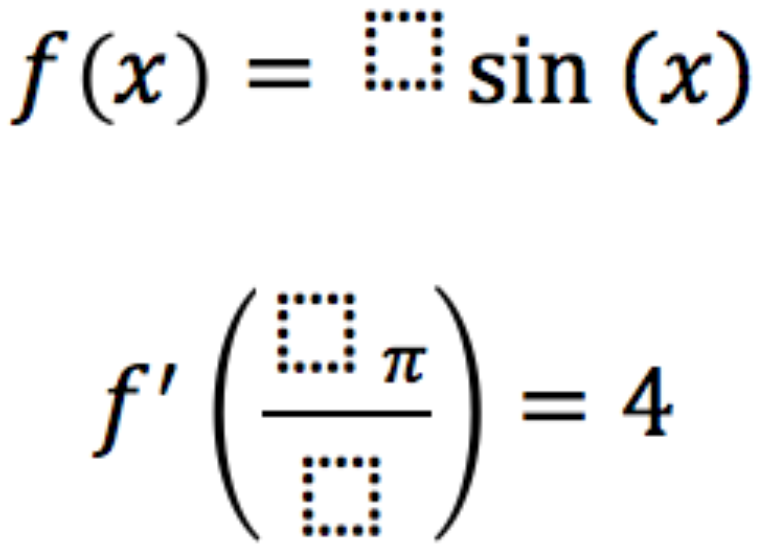# Derivative of Trig Functions 1

Directions: Fill in the boxes below using the digits 1 to 9, at most one time each, to make as many possible solutions as you can.### Hint

What values of trig functions give “easy to work with” solutions?

One possible answer: 4sin(x) and f'(6pi/3)

Source: Chris Luzniak

## Fundamental Theorem Of Calculus

Directions: Using the digits 0 to 9 at most one time each, place a digit …

1.2.•